##CS 662 Theory of Parallel Algorithms Odd-Even Sort

[To Lecture Notes Index]
San Diego State University -- This page last updated February 20, 1996, 1996## References

Akl, The Design and Analysis of Parallel Algorithms, section 4.3
Rescheduling Principle

A parallel machine model satisfies the rescheduling principle (or slow down principle) if given an algorithm with time complexity T on P processors and given any s, 1 <= s <= p the algorithm can be run in s*T time using at most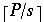processors.

Models with uniform cost per access of shared memory satisfy the rescheduling principle.

proof: Draw picture and wave hands

Odd-Even Transposition Sort

Assume each processor PJ contains one element xJ, for J = 1, 2, ..., N

Odd-Even Transposition Sort
for K = 1 to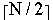do
for I = 1, 3, 5, ...,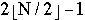do in parallel
if xI> xI+1 then swap(xI,xI+1)
end for
for I = 2, 4, 6, ...,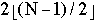do in parallel
if xI> xI+1 then swap(xI,xI+1)
end for
end for

A Better Odd-Even Transposition Sort

Assume we have P processors and N items to sort

Each processor has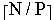items

Pad the last processor with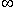to insure it hasitems

Let SJ be the items in processor PJ

```Odd-Even Transposition Sort
for K = 1 to P do in parallel
QuickSort(SK)
end for
for K = 1 to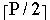do
for I = 1, 3, 5, ...,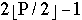do in parallel
(s1, s2, s3, ...,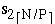) = sequential merge(SI, SI+1)
SI = (s1, s2, s3, ...,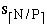)
SI+1 = (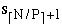,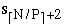...,)
end for

for I = 2, 4, 6, ...,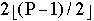do in parallel
(s1, s2, s3, ...,) = sequential merge(SI, SI+1)
SI = (s1, s2, s3, ...,)
SI+1 = (,...,)
end for
end for
```